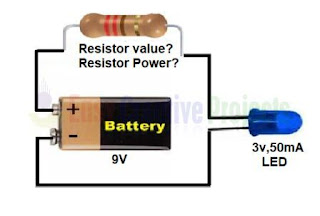# How to find perfect resistor value and power for any project

How to find perfect resistor value and power for any project with the help of simple example and calculation using ohm law.

## How to find perfect resistor for any project?

We learn it with a simple example that describe how to calculate right resistance value and power for your project using ohm law.

#### Example:I have one led 3v and 50mA. I want to run it on 9v battery. so what is the perfect resistor for my project?

1st we need to find resistor value. we easily find resistor value using ohms law.
ohms law says

R = V/I

and we have 2 voltage
1. VS (Input Voltage, or Source Voltage)
2. VL (LED Voltage or Load Voltage)
R=VS - VL/I

So

Vs = 9V (Battery power)
VL = 3v (LED Voltage)
I  = 50mA (or 0.05 ampere)
R = ?
after putting values

R =  9-3/0.05
R = 6/0.05
R =  120 ohm

mean if we use 120 ohm resistor then we easily run led with 9v battery.
but how to find how many power Resistor need?
we know Joule's Law power formula is

P=I2R

I
=  0.05Amp
R = 120 ohm
P = ?
After putting value

P= (0.05)x 120
P=0.0025 x 120
P=0.3W

so
we need 120 ohm and 0.3 watt power resistor.
using this example you can easily find any resistor value for your project.
if same value not available then you can use nearest value.
Labels: